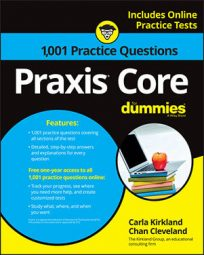##### Praxis CoreFinding the prime factors of a number is a really useful skill to have—not lost-in-the-wilds or trapped-in-a-warehouse-full-of-ninjas useful, but certainly handy for the Praxis Core exam.

To help you prepare, the following practice questions ask you to choose the number that is not a factor of another number, and then to find the greatest common factor of two numbers.

## Practice questions

1. Which of the following is NOT a factor of 42?

A. 7 B. 6 C. 14 D. 21 E. 18

2. What number is the greatest common factor of 28 and 72?

A. 4 B. 1 C. 7 D. 2 E. 14

1. The correct answer is Choice (E).

You can find all the factors of 42 by getting its prime factorization—the prime numbers you multiply by each other to get 42—and then finding all the products of the combinations of the factors. In this case, each prime factor is a factor only once:

The only answer choice that isn't a product of a combination of prime factors of 42 is Choice (E), 18. Choice (A) is a prime factor of 42,

2. The correct answer is Choice (A).

You can use the prime factorizations of 28 and 72 to determine their factors:

• Factors of 28: 1, 2, 4, 7, 14, 28
• Factors of 72: 1, 2, 3, 4, 6, 8, 9, 12, 18, 24, 36, 72

The largest number that is a factor of both 28 and 72 is 4, so it's their greatest common factor.